+
Summation Notation
Solving Linear Equations and Inequalities
0
of 0 possible points

# Summation Notation

Author: Sophia Tutorial
##### Description:

Evaluate a series using summation notation.

(more)

Sophia’s self-paced online courses are a great way to save time and money as you earn credits eligible for transfer to many different colleges and universities.*

No credit card required

46 Sophia partners guarantee credit transfer.

299 Institutions have accepted or given pre-approval for credit transfer.

* The American Council on Education's College Credit Recommendation Service (ACE Credit®) has evaluated and recommended college credit for 33 of Sophia’s online courses. Many different colleges and universities consider ACE CREDIT recommendations in determining the applicability to their course and degree programs.

Tutorial
what's covered
1. Sequences and Series
2. Summation Notation
3. Evaluate a Series using Summation Notation

# 1. Sequences and Series

A sequence is a set of numbers in a particular order. For example, {4, 7, 10, 13, 16} is a sequence. It has elements or terms in curly braces (which define it as a set), and the terms are in numerical order (increasing in value). One application of sequences is to add the terms together. We call this a series. Let's review these terms and their definitions:

terms to know
Sequence
A set of numbers in a particular order.
Series
The sum of the first n terms in a sequence.

To evaluate the series of the sequence {4, 7, 10, 13, 16} we simply add the numbers together. The series evaluates to 50.

# 2. Summation Notation

Summation notation is a way to write the sum of sequences. Let's us the sequence {3, 5, 7, 9, 11} to write the series in summation notation: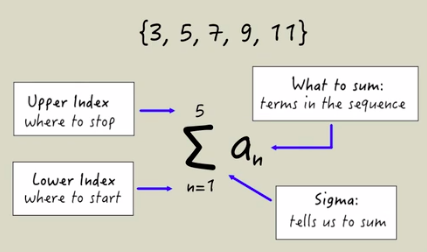This notation tells us to sum the values of terms in a sequence, starting with the first term and ending with the fifth term. How was this information drawn out from this notation? The big E-like symbol is the Greek letter sigma. This is what tells us to sum. What exactly are we summing? That's written directly to the right of sigma. an represents the value of the n-th term to a sequence. Finally, we see expressions above and below sigma. Below is the lower index, which tells us where to start. In this case, the lower index is 1. The 5 above is the upper index, and tells us where to stop summing. So this is telling us to evaluate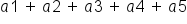, where each of these terms represents the values of the first, second, third, fourth, and fifth terms of a sequence, respectively.

term to know
Summation notation
an expression of a series, using the Greek letter sigma, and a lower and upper index to indicate the first and last terms of the sum.

# 3. Evaluating a Series using Summation Notation

Next, let's practice using a defined sequence and summation notation to evaluate a series.

Consider this example sequence {12, 8, 4, 0, -4, -8, ...}:

Notice, however, that this is now an infinite sequence, meaning that more terms follow 8. How can we interpret what we are asked to sum from the following notation: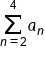We should first note what this is asking us to sum. This is telling us to add values of terms in a sequence. Our sequence is defined as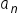, so this is asking us to add together terms in that particular sequence. Next, we should examine the lower index, which tells us what term is the start to our sum, as well as the upper limit, which tell us which term will end the sum. In this case, the lower limit is 2 and the upper limit is 4. The notation tells us to add together the second through fourth terms of the sequence. The second term is 8, the third term is 4, and the fourth term is 0.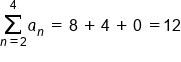This would be 8 + 4 + 0 = 12 as the value of the series.

summary
When defining sequences and series, we can say that a sequence is a set of numbers in a particular order and a series is the sum of the first n terms in a sequence. Summation notation is an expression of a series from a lower index term up to and including an upper index term. When evaluating a series using summation notation, it is important to note what it is asking us to sum.

Terms to Know
Sequence

a set of numbers in a particular order

Series

the sum of the first nth terms in a sequence

Summation Notation

an expression of a series, using the Greek letter sigma, and a lower and upper index to indicate the first and last terms of the sum

Rating